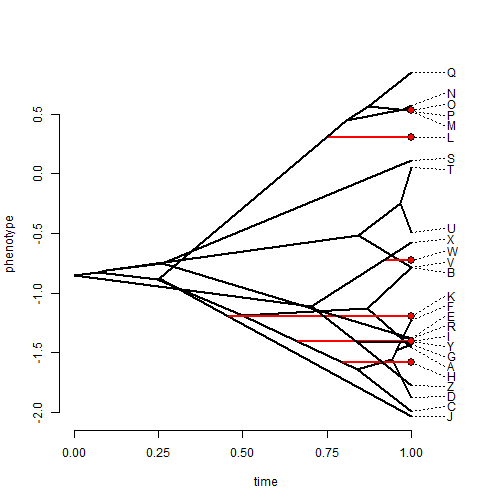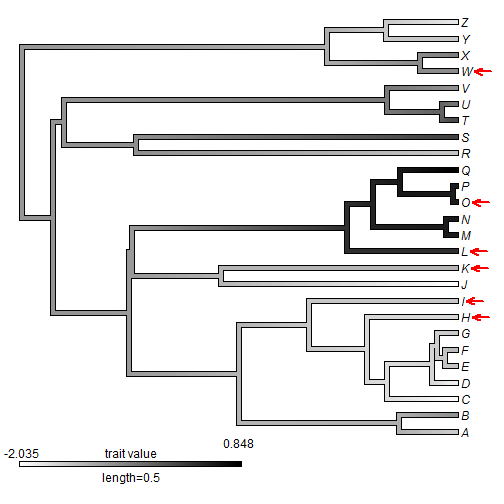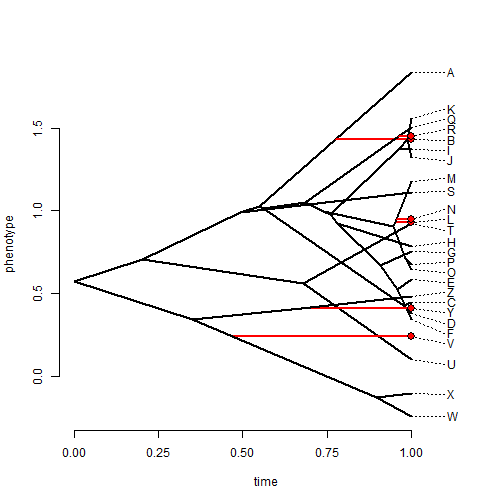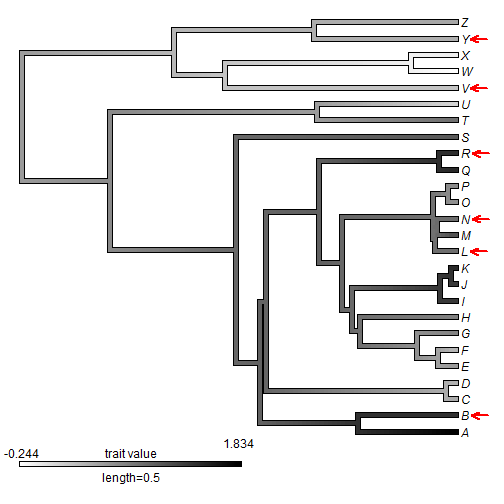## Thursday, October 20, 2016

### Estimation of missing tip states under Brownian motion using likelihood

As I have noted previously, it is possible (and relatively straightforward) to reconstruct missing tip values under Brownian motion by jointly maximizing the likelihood of internal and (missing) terminal states using likelihood.

This is implemented in the phytools function `anc.ML`.

One attribute of these reconstructed values is that they will precisely match the reconstructed ancestral node state of their immediate parent. This is totally unsurprising because under Brownian motion because the expected change over any time period is zero (with variance equal to the product of the Brownian rate, σ2, and the edge length).

We can visualize this phenomenon using both `phenogram` and `contMap`. Here's what I mean.

First, simulate a tree & some data:

``````library(phytools)
tree<-pbtree(n=26,tip.label=LETTERS,scale=1)
x<-fastBM(tree)
``````

Subsample the data so there is missing information for some tips:

``````xp<-sample(x,20)
``````

Fit our ancestral states & missing terminals using ML:

``````fit<-anc.ML(tree,xp)
fit
``````
``````## Ancestral character estimates using anc.ML under a BM model:
##        27        28        29        30        31        32        33
## -0.851047 -0.824691 -0.884692 -1.184654 -1.128078 -1.402134 -1.580146
##        34        35        36        37        38        39        40
## -1.642514 -1.551363 -1.477286 -1.374941 -0.878525 -1.194390  0.306645
##        41        42        43        44        45        46        47
##  0.450288  0.539937  0.568322  0.527984 -0.806273 -0.753233 -0.514332
##        48        49        50        51
## -0.251404 -1.112156 -0.722011 -1.412652
##
## Fitted model parameters & likelihood:
##      sig2 log-likelihood
##  0.468265       5.497266
##
## R thinks it has found the ML solution.
``````

Note that reconstructed missing tip values match their parents (to fairly high numerical precision - this is obtained via numerical optimization, remember):

``````tips<-sapply(names(fit\$missing.x),function(x,y)
which(y==x),y=tree\$tip.label)
library(phangorn)
parents<-sapply(tips,Ancestors,x=tree,type="parent")
X<-rbind(fit\$missing.x,fit\$ace[as.character(parents)])
rownames(X)<-c("daughter","parent")
X
``````
``````##                  H         I         K         L         O          W
## daughter -1.580114 -1.402163 -1.194513 0.3066923 0.5279829 -0.7220155
## parent   -1.580146 -1.402134 -1.194390 0.3066445 0.5279840 -0.7220109
``````

Now let's visualize it - first with `phenogram`:

``````x.all<-c(xp,fit\$missing.x,fit\$ace)
tree<-paintBranches(tree,tips,"2")
phenogram(tree,x.all)
nodelabels(node=tips,pie=rep(1,length(fit\$missing.x)),
cex=0.5)
``````or with `contMap`:

``````obj<-contMap(tree,x.all,method="user",anc.states=fit\$ace,
plot=FALSE)
obj<-setMap(obj,colors=c("white","black"))
plot(obj)
arrl=0.04,hedl=0.02,col="red")
``````Finally, let's repeat the whole thing just to show it wasn't a fluke:

``````tree<-pbtree(n=26,tip.label=LETTERS,scale=1)
x<-fastBM(tree)
xp<-sample(x,20)
fit<-anc.ML(tree,xp)
tips<-sapply(names(fit\$missing.x),function(x,y)
which(y==x),y=tree\$tip.label)
parents<-sapply(tips,Ancestors,x=tree,type="parent")
X<-rbind(fit\$missing.x,fit\$ace[as.character(parents)])
rownames(X)<-c("daughter","parent")
X
``````
``````##                 B         L         N        R         V         Y
## daughter 1.430460 0.9306579 0.9480089 1.449290 0.2423973 0.4115517
## parent   1.430375 0.9306499 0.9480131 1.449279 0.2425937 0.4116535
``````
``````x.all<-c(xp,fit\$missing.x,fit\$ace)
tree<-paintBranches(tree,tips,"2")
phenogram(tree,x.all)
nodelabels(node=tips,pie=rep(1,length(fit\$missing.x)),
cex=0.5)
````````````obj<-contMap(tree,x.all,method="user",anc.states=fit\$ace,
plot=FALSE)
obj<-setMap(obj,colors=c("white","black"))
plot(obj)Recently, it was reported to me that for some empirical dataset this has not been observed to be the case. The most likely explanation for this is non- convergence of the ML optimization by `anc.ML`. This can be ameliorated by increasing `maxit`, but this may not guarantee convergence on relatively large trees. I'm sure that a better solution exists, unfortunately I have not worked on this in a bit.